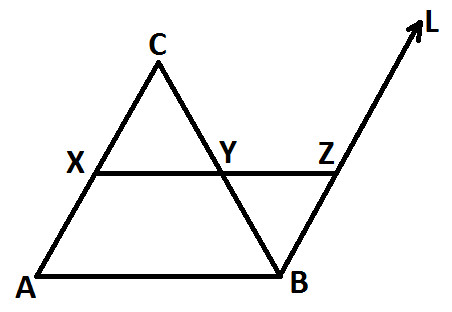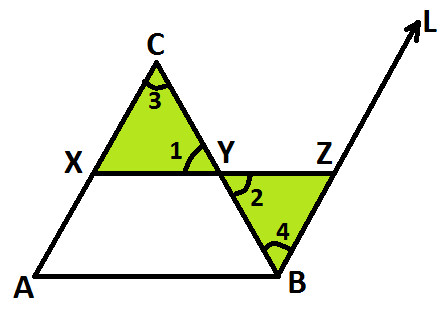Arithmetic
Arithmetic Progression
Associative Property
Averages
Brackets
Closure Property
Commutative Property
Conversion of Measurement Units
Cube Root
Decimal
Divisibility Principles
Equality
Exponents
Factors
Fractions
Fundamental Operations
H.C.F / G.C.D
Integers
L.C.M
Multiples
Multiplicative Identity
Multiplicative Inverse
Numbers
Percentages
Profit and Loss
Ratio and Proportion
Simple Interest
Square Root
Unitary Method
Algebra
Cartesian System
Order Relation
Polynomials
Probability
Standard Identities & their applications
Transpose
Geometry
Basic Geometrical Terms
Circle
Curves
Angles
Define Line, Line Segment and Rays
Non-Collinear Points
Parallelogram
Rectangle
Rhombus
Square
Three dimensional object
Trapezium
Triangle
Trigonometry
Trigonometry Ratios
Data-Handling
Arithmetic Mean
Frequency Distribution Table
Graphs
Median
Mode
Range

Videos
Solved Problems
Home >> Triangle >> Properties >> Mid point property of Triangle >>

## Mid point property of Triangle

 Sum of Two Sides Angle Sum Property Angles opposite to equal sides of triangle are equal Angle opposite to longer side is greater Pythagoras Theorem Exterior Angle Property of a Triangle Mid point property of Triangle Triangles on same base & between same parallel lines

 What is Mid-Point Property of Triangle ? Mid point property of triangle says : The line segment joining the mid-points of two sides of a triangle, is parallel to the third side Let's understand this with the help of following diagram:ABC is a triangle X and Y are the mid points of sides AC and BC respectively XY is the line segment which joins the mid-points of AC and BC sides Now, Mid point property of triangle says that the line segment joining the mid-points of two sides of a triangle, is parallel to the third side. So in context of the above diagram, we get: XY // AB How to prove the mid-point property of triangle: Before you understand how to prove this property, you are advised to read: What is Transversal ? What are Alternative Interior Angles of Transversal ? What are Vertically Opposite Angles ? What is Congruent Triangle ? What are the Rules of Congruency ? Observe the below diagram:ABC is a triangle X and Y are the mid points of sides AC and BC respectively, so we get: AX = XC and CY = YB ..... (statement 1) Now, from vertex B draw a line BL parallel to AC (as shown below)Extend line XY such that it meets BL at Z (as shown below):Now, BL // AC, so we can also say that: AX // BZ ..... (statement 2) Take two Triangles XYC and YZB (as highlighted in below diagram):In △ CXY and △ YZB ∠ 1 = ∠ 2 (vertically opposite angles) CY = YB (proved in statement 1) ∠ 3 = ∠ 4 (Alternative interior angles - AC // BL, CB is a transversal) Therefore, by ASA rule of Congruency, we get: △ CXY ≅ △ YZB Since, △ CXY ≅ △ YZB, so we get: XC = BZ (because corresponding sides of congruent triangles are equal) ..... (Statement 3) From statement 1: AX = XC From statement 3: XC = BZ So, from statement 1 and 3, we get: AX = BZ ..... (statement 4) Now, observe Quadrilateral ABZX (as highlighted in below diagram):From statement 2 and 4, we get: AX // BZ AX = BZ Since AX and BZ are opposite side, so we get: Quadrilateral ABZX is a parallelogram (because if opposite side of a quadrilateral are equal and parallel, then the quadrilateral is a parallelogram) Now, in parallelogram ABZX, we get: XZ // AB (because in parallelogram opposite side are parallel) Or we can also write it as: XY // AB Hence proved, Mid point property of triangle : The line segment joining the mid-points of two sides of a triangle, is parallel to the third side Now, since Mid point property is proved, so we get converse of this property i.e.: The line drawn through the mid-point of one side of a triangle, parallel to another side bisects the third side.

Copyright@2022 Algebraden.com (Math, Algebra & Geometry tutorials for school and home education)